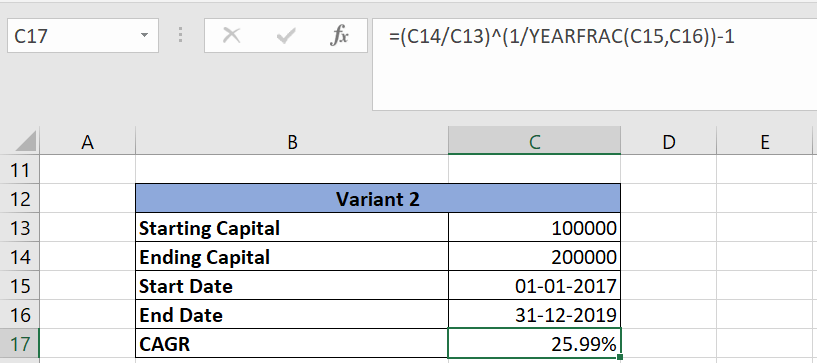# How to calculate CAGR in Excel Sheet?

When it comes to complex financial calculations, probably “Excel” is the first tool that comes to your mind. With technology advancements, there are several other tools and softwares that have evolved but nothing can surpass excel in terms of simplicity as well as versatility. In this post, we’ll learn how to calculate CAGR in excel sheet. This is an important parameter to evaluate the performance of any algorithmic trading system.

Let’s first understand what is CAGR-

## CAGR Definition

CAGR stands for compounded annual growth rate. It is the rate of return required to grow your investment from a given starting capital to ending capital, assuming that the profit at the end of each year is re-invested.

It is calculated using the below formula

CAGR= (EC/SC)1/n -1

Where

SC – Starting Capital

EC – Ending Capital

N – Number of years

For example- if you have a trading system that grows your capital from 100K to 200K in 3 years. Then

CAGR = (200000/100000)1/3 -1 = 0.2599 or 25.99%

CAGR is usually represented in % terms and used to compare the performance of trading systems. It is readily available in the backtesting report of modern charting platforms like Amibroker.

## Calculate CAGR is Excel Sheet

If you understand the formula above to calculate CAGR, calculating it in an Excel sheet would be a cakewalk.

There may be two variants depending on the inputs available:

Variant 1: You know the starting capital, ending capital and number of yearsHere CAGR is calculated using the formula =(C7/C6)^(1/C8)-1

Cell C7 contains ending capital, C6 contains starting capital and C8 contains number of years

Variant 2: You know the starting capital, ending capital, start date, and end dateHere CAGR is calculated using the formula =(C14/C13)^(1/YEARFRAC(C15,C16))-1

Cell C14 contains ending capital, C13 contains starting capital, C15 is the start year and C16 is the end year.

The number of years is internally calculated using the formula YEARFRAC